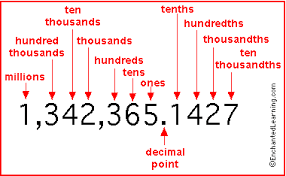# How do you change two and one half into a decimal?

Are you turning a fraction into one? If so then you can only turn fractions that are 1 in 10 or 100. So how do you turn 1 half into a tenth? Make it 5 tenths! Which would be 5 over 10.

Now the 2 is a whole number right? Then to start the decimal do 2. Now the 10 plays a good role in decimals if it's a 10 put the number over the 10 which is 5 next to the dot in 2.

To get 2.5 let's say it had to be 5 hundredths this means you would do 2.05 but in this case the answer is 2.5 if I am correct you're welcome because I am only in 5th grade.

1 Person thanked the writer.
Ash Rett commented
I've been in three types of gifted and talented 2 of them were for math

Converting whole numbers and fractions into whole numbers with decimals is quite straightforward.

In this instance, two and a half converts into 2.5. The half goes behind the decimal point as it is smaller than the number in the 'singles' column.As you can see, the .5 goes into the first column behind the decimal point, the 'tenths' column. Therefore, it is the same as five-tenths, which reduced to its lowest common denominators, gives us one-half, which is what we started with.

The 2 in 2.5, as a single whole number, belongs in the first column to the left of the decimal point, the singles column.

When thinking about a decimal number, it can be easier to think of it as a whole number with a fraction. For example, the number 3.75 as a fraction would be three and three-quarters.

In this case, we go .75 as a hundredth of a whole number, as the last digit sits in the hundredths column, behind the decimal point. Three-quarters of a hundred is 75, so we know .75 is the correct way to express three-quarters as a decimal.

For more Blurtit help with whole numbers and decimals, have a look at How Do I Change 2 3/4 Into A Decimal? and How do you change fractions and percentages into decimals? Or, you can watch this useful YouTube film on the subject:

thanked the writer.